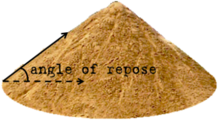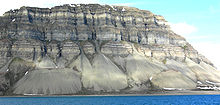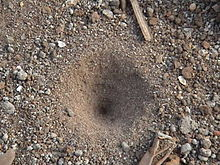Angle of repose

﻿
Angle of reposeAngle of repose

The angle of repose or, more precisely, the critical angle of repose, of a granular material is the steepest angle of descent or dip of the slope relative to the horizontal plane when material on the slope face is on the verge of sliding. This angle is in the range 0°–90°.

When bulk granular materials are poured onto a horizontal surface, a conical pile will form. The internal angle between the surface of the pile and the horizontal surface is known as the angle of repose and is related to the density, surface area and shapes of the particles, and the coefficient of friction of the material. Material with a low angle of repose forms flatter piles than material with a high angle of repose.

The term has a related usage in mechanics, where it refers to the maximum angle at which an object can rest on an inclined plane without sliding down. This angle is equal to the arctangent of the coefficient of static friction μs between the surfaces.

Applications of theoryTalus cones on north shore of Isfjord, Svalbard, Norway, showing angle of repose for coarse sediment.

The angle of repose is sometimes used in the design of equipment for the processing of particulate solids. For example, it may be used to design an appropriate hopper or silo to store the material, or to size a conveyor belt for transporting the material. It can also be used in determining whether or not a slope (of a stockpile, or uncompacted gravel bank, for example) will likely collapse; the talus slope is derived from angle of repose and represents the steepest slope a pile of granular material will take. This angle of repose is also crucial in correctly calculating stability in vessels.

It is also commonly used by mountaineers as a factor in analysing avalanche danger in mountainous areas.

Measurement

There are numerous methods for measuring angle of repose and each produces slightly different results. Results are also sensitive to the exact methodology of the experimenter. As a result, data from different labs are not always comparable. One method is the triaxial shear test, another is the direct shear test.

If the coefficient of static friction is known of a material, then a good approximation of the angle of repose can be made with the following function. This function is somewhat accurate for piles where individual objects in the pile are minuscule and piled in random order.$\tan{(\theta)} \approx \mu_s\,$

Where:

• μs is the coefficient of static friction
• θ is the angle of repose
Procedure to Measure and Calculate the Angle of Repose
1. Pour the dry sand into a pile on a level surface allowing it to build a pile from the top. This will result in a pile with a relatively circular base, making measurement easier.
2. Using the ruler and a tape measure, measure the height (h) of the pile of sand from the peak to the ground. Stand the ruler next to the pile so it can be read easily. Extend the tape measure carefully to the top of the pile without disturbing the pile and allow the other end of the tape measure to intersect the ruler. While keeping the tape measure level, observe the intersection of the tape measure with the ruler. Write the value on the paper. (For example: if the height of the pile is 12 units, write h = 12)
3. Using the tape measure, measure the horizontal distance (d) from the middle of the pile to the edge. Place the tape measure on the ground beside the pile. Line up one end with one side of the pile and extend the tape measure to the other end of the pile. Write the value on the paper and divide by 2. This will give you the distance from the centre of the pile to the edge. (For example: If the total distance on tape measure from one end of the pile to the other is 30 units, divide by 2 to get 15. d= 15)
4. The equation for calculating the angle of repose is tan-1(h/d) . Using your scientific calculator, multiply height (h calculated in Step 2) by 2 and divide this value by the distance (d calculated in Step 3). Then, hit the inverse tangent key (or tan-1) and the answer just calculated. This will give you the angle of repose, α.
5. Place the protractor on the level surface next to the pile of sand. Using the ruler, create a straight line from the peak of the sand pile down the slope. Read the angle of repose value (α) and write the value on the paper.
6. Compare the calculated angle of repose from Step 4 and the measured angle of repose from Step 5. If the values are not within 1 degree of each other, repeat Step 5.

Exploitation by antlion and wormlion (Vermileonidae) larvaeSand pit trap of the antlion

The larvae of the antlions and the unrelated wormlions Vermileonidae trap small insects such as ants by digging conical pits in loose sand, such that the slope of the walls is effectively at the critical angle of repose for the sand. They achieve this by flinging the loose sand out of the pit and permitting the sand to settle at its critical angle of repose as it falls back. Thus, when a small insect, commonly an ant, blunders into the pit, its weight causes the sand to collapse below it, drawing the victim toward the center where the predator that dug the pit lies in wait under a thin layer of loose sand. The larva assists this process by vigorously flicking sand out from the center of the pit when it detects a disturbance. This undermines the pit walls and causes them to collapse toward the center. The sand that the larva flings also pelts the prey with so much loose, rolling material as to prevent it from getting any foothold on the easier slopes that the initial collapse of the slope has presented. The combined effect is to bring the prey down to within grasp of the larva, which then can inject venom and digestive fluids.

Methods in Determining the Angle of Repose

Tilting Box Method This method is appropriate for fine-grained, non-cohesive materials, with individual particle size less than 10 mm. The material is placed within a box with a transparent side to observe the granular test material. It should initially be level and parallel to the base of the box. The box is slowly tilted at a rate of approximately .3 degrees/second. Tilting is stopped when the material begins to slide in bulk, and the angle of the tilt is measured.

Fixed Funnel Method The material is poured through a funnel to form a cone. The tip of the funnel should be held close to the growing cone and slowly raised as the pile grows, to minimize the impact of falling particles. Stop pouring the material when the pile reaches a predetermined height or the base a predetermined width. Rather than attempt to measure the angle of the resulting cone directly, divide the height by half the width of the base of the cone. The inverse tangent of this ratio is the angle of repose.

Revolving Cylinder Method The material is placed within a cylinder with at least one transparent face. The cylinder is rotated at a fixed speed and the observer watches the material moving within the rotating cylinder. The effect is similar to watching clothes tumble over one another in a slowly rotating clothes dryer. The granular material will assume a certain angle as it flows within the rotating cylinder. This method is recommended for obtaining the dynamic angle of repose, and may vary from the static angle of repose measured by other methods. When describing the angle of repose for a substance, always specify the method used.

Angle of Repose of various materials

Here is a list of various materials and their angle of repose. All measurements are approximated and not exact.

Material (condition) Angle of Repose (degrees)
Ashes 40°
Asphalt (crushed) 30–45°
Bark (wood refuse) 45°
Bran 30–45°
Chalk 45°
Clay (dry lump) 25–40°
Clay (wet excavated) 15°
Clover seed 28°
Coconut (shredded) 45°
Coffee bean (fresh) 35–45°
Earth 30–45°
Flour (wheat) 45°
Granite 35–40°
Gravel (loose dry) 30–45°
Gravel (natural w/ sand) 25–30°
Malt 30–45°
Sand (dry) 34°
Sand (water filled) 15–30°
Sand (wet) 45°
Wheat 28°

The angle of repose plays a part in several topics of technology and science, including:

Wikimedia Foundation. 2010.

Look at other dictionaries:

• Angle of repose — Repose Re*pose , n. [F. repos. See {Repose}, v.] 1. A lying at rest; sleep; rest; quiet. [1913 Webster] Shake off the golden slumber of repose. Shak. [1913 Webster] 2. Rest of mind; tranquillity; freedom from uneasiness; also, a composed manner… …   The Collaborative International Dictionary of English

• angle of repose — n. the maximum angle of slope at which sand, loose rock, etc. will remain in place without sliding, as on a hillside …   English World dictionary

• angle of repose — trinties kampas statusas T sritis fizika atitikmenys: angl. angle of friction; angle of repose; limiting angle; limiting angle of friction vok. Gleitwinkel, m; Grenzwinkel, m; Grenzwinkel der Reibung, m; Reibungswinkel, m rus. предельный угол, m; …   Fizikos terminų žodynas

• angle of repose — n the greatest angle, measured from horizontal, attained by a coal so that material on the sides of top of the inverted cone or trench created in car top sampling remains stable, that is, will not shift or slide. Coal size and moisture content… …   Coke&Coal Terminology

• angle of repose — 1. physics : the angle that the plane of contact between two bodies makes with the horizontal when the upper body is just on the point of sliding : the angle whose tangent is the coefficient of friction between the two bodies 2. or angle of rest …   Useful english dictionary

• angle of repose — Geol. the maximum slope, measured in degrees from the horizontal, at which loose solid material will remain in place without sliding. Cf. angle of slide. [1875 80] * * * …   Universalium

• angle of repose —    The maximum angle of slope (measured from a horizontal plane) at which loose, cohesionless material will come to rest.    GG …   Glossary of landform and geologic terms

• angle of repose — noun the steepest angle at which a sloping surface formed of loose material is stable …   English new terms dictionary

• angle of repose — noun Geology the maximum angle at which unconsolidated material, such as scree, will remain stable …   Australian English dictionary

• angle of repose —    The natural slope of unsupported granular material  …   Lexicon of Cave and Karst Terminology